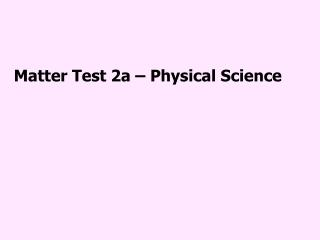# Matter Test 2a – Physical Science - PowerPoint PPT PresentationDownload PresentationMatter Test 2a – Physical Science

Matter Test 2a – Physical Science
Download Presentation## Matter Test 2a – Physical Science

- - - - - - - - - - - - - - - - - - - - - - - - - - - E N D - - - - - - - - - - - - - - - - - - - - - - - - - - -
##### Presentation Transcript

1. Matter Test 2a – Physical Science

2. 2) If I take away 450 Joules of heat energy to 600 mL of water at 0ºC, what will happen to the water molecules according to the Kinetic Theory of Matter?

3. 3) Explain what temperature is.

4. 4) 15.60 °C = __________ K 5) -73 °C = __________ K 6) 9 K = _____________°C 7) 98.3 K = ________________°C

5. What state of matter can be massed in grams but since its volume is indefinite, it can’t truly be measured with a graduated cylinder? Indefinite = not definite, changing

6. 9) The electrons flowing through the gases in a fluorescent light bulb heats the gases up to thousands of degrees Celsius and turns the gases into ______________________.

7. 10) In the graph shown above, what state of matter is the “piece of matter” at if 430 Joules of energy is applied to it?

8. 11) If the graph shown above is for pure water (H2O) as the matter sample, about how many Joules of energy would be required to change it completely to a liquid?

9. 12) If the graph shown above is for pure water (H2O) as the matter sample, at what temperature Celsius would the sample be after ading 800 Joules of energy?

10. 13) If the graph shown above is for pure water (H2O) as the matter sample, what state of matter is probably not shown on the graph?

11. Explain why the pressure becomes greater in the box pictured above when the box becomes smaller, according to the Kinetic Theory of Matter?

12. 15) In which of the states of matter depicted above are Intermolecular forces stronger than the kinetic force of the atoms?

13. 16) Would it be easier for large particles to go from the right state of matter to the left state of matter or would it be easier for small particles to go from the right state of mater to he left state of matter?

14. 17) The two boxes shown above have exactly the same pressure. In which box are the particles traveling faster?

15. 18) In the illustration above of our Sun, what state of matter are we seeing?

16. 19) In the illustration above, according to the Kinetic Theory of Matter, what would you have to do to completely stop the particles from moving?

17. 20) According to the Kinetic Theory of Matter, the atoms and the molecules around us act as discrete _____________________. Discrete = individually separate and distinct

18. 22) In what state of matter would 100 grams of sulfur contain the least heat energy?

19. The three assumptions that are made in the Kinetic Theory of Matter are: 23) All matter is made of _________________. 24) These are in constant ______________ 25) All collisions are perfectly __________________ (molecules do not lose energy in collisions Word Bank: Aligned Angled Decay Elastic Molecules Motion Particles Quarks Strings Touch

20. 26) Absolute zero on the Celsius scale is _____________________.

21. 27-28) List two types of things found in the real world that act like the ideal particles in the Kinetic Theory of Matter.

22. 29) Explain what temperature is.

23. 30) Explain what heat is.